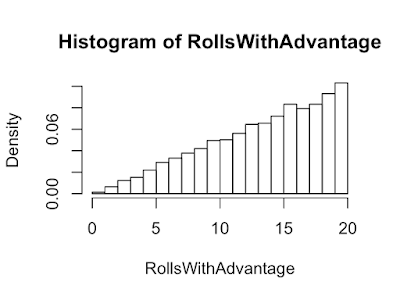## Wednesday, June 24, 2020

#### D20 and Random Events

In the game Dungeons and Dragons, the success or failure of an event is determined by rolling a 20 sided die (D20): higher is better. If you need to roll 11 or higher you have a 50% chance of success. If another event requires 10 or better you now have a 55% chance for success. Each point the roll goes up or down is worth 5%. Often times, rolling a 20 is a critical success and a 1 is a critical failure; critical means it's extra good/bad.

Sometimes things are really going your way and you roll with advantage. Sometimes things are not in your favor and you roll with disadvantage. When rolling with advantage, roll two dice and pick the higher. When rolling with disadvantage, roll two dice and pick the lower. How do advantage and disadvantage affect the chance of getting a 20 or 1?

When rolling without advantage or disadvantage, the probability of getting a 20 or a 1 is 1/20 = 0.05 or 5%. The probability of not getting a twenty 1 - 1/20 = 19/20.

```P20    <- 0.05
Pnot20 <- 1-P20
```

When you have advantage, to not get a 20, you have to not roll a 20 twice. The probability of getting a 20 is (1 - the proability of not getting a 20 twice), and as you can see below, is almost 10%. Advantage about doubles you chance of getting a 20. But you probability guessed that since you're rolling twice. :) The chance of getting a 1 is 0.05^2=0.0025, almost 0!

```Pnot20*Pnot20
```
```##  0.9025
```
```1 - Pnot20*Pnot20
```
```##  0.0975
```

How will advantage and disadvantage affect the average roll? This time let's estimate the answer using a simulation. A 1000 trials will give a good estimate.

```Trials <-10000
```

Below we simulate rolling two D20s

```set.seed(1)
x <- sample(1:20,Trials,replace = TRUE)
y <- sample(1:20,Trials,replace = TRUE)
```

When we have advantage, roll two D20 and pick the max. With disadvantage roll two D20 and pick the min.

```RollsWithAdvantage <- apply(cbind(x,y), 1,max)
```

```Advantage
```
```##  13.8863
```
```mean(1:20)
```
```##  10.5
```
```Disadvantage
```
```##  7.1287
```

Below are 3 figures with histograms. The first histogram is made with 10,000 simulated D20 advantage rolls. Advantage moves a lot of probability to the right. The second histogram is made using 10,000 simulated D20 regular rolls. The histogram is approximately flat and each number is at about 0.05% and that's what we calculated. The last histogram is made with 10,000 simulated D20 disadvantage rolls. Disadvantage moves an equal amount to the left.The probability of getting 11 or better with advantage is 1- probability of getting 10 or less twice. Rolling with advantage moves a 50% chance to a 75% chance!

```p10orLess <- 0.5
1 - p10orLess^2
```
```##  0.75
```

## Saturday, June 20, 2020

### Complex Normal Samples In R

#### Normal Samples

If we want 10 samples from a Gaussian or normal random process with variance 4 can use rnorm(10,sd=2). Remember the standard deviation (sd) is the square root of the variance.

```set.seed(2)
x <- rnorm(10,sd=2)
x
```
```##   -1.7938291  0.3696984  3.1756907 -2.2607513 -0.1605035  0.2648406
##    1.4159095 -0.4793960  3.9689479 -0.2775740
```
```var(x)
```
```##  3.880803
```

The var() function produces an estimate of the variance, if we want a better estimate we need more samples.

```var(rnorm(1000,sd=2))
```
```##  4.105966
```

#### Complex Normal Samples

If we are using base R and want complex normal (CN) samples, we need to write our own function. When the signal processing literature refers to CN they are usually referring to circularly-symmetric CN. Circularly-symmetric means the samples are independent and their mean is 0.

The function produces N CN samples with variance v. The real and imaginary parts are independent, because they are produced by different calls to rnorm(). Let x,y be independent. The var(ax) = a^2var(x) and var(x+y)=var(x)+var(y). So, if we want a variance of 1 would have to start a variance of sqrt(1/2).

```makeCN <- function(N,v=1) {(sqrt(v/2))*rnorm(N) + (sqrt(v/2))*1i*rnorm(N)}
makeCN(10)
```
```##    0.0023376-0.2079938i  0.7613032+0.6053620i  0.3946671-0.4049715i
##    0.4892950-0.1207824i  0.4651165-0.2871364i -0.2312504+0.9408834i
##   -0.2153405-0.9648887i -1.0994866+1.0119199i  1.0396552+0.7824796i
##   0.1147878+0.9059002i
```

If we want to check the variance, we can't use var() directly.

```var(makeCN(10))
```
```## Warning in var(makeCN(10)): imaginary parts discarded in coercion
```
```##  0.6039204
```

But the real and imaginary parts are independent, so we can calculate the variance separately.

```z <- makeCN(10)
var(Re(z)) + var(Im(z))
```
```##  0.5623849
```

To make this easier, we can create a function to find the variance.

```varComplex <- function(z) var(Re(z)) + var(Im(z))
```

To get a good estimate we need a-lot of samples.

```varComplex(makeCN(1000))
```
```##  1.016615
```

Let's set the variance to 2 and then estimate the variance of the samples.

```varComplex(makeCN(1000,v=2))
```
```##  1.925119
```

Success!